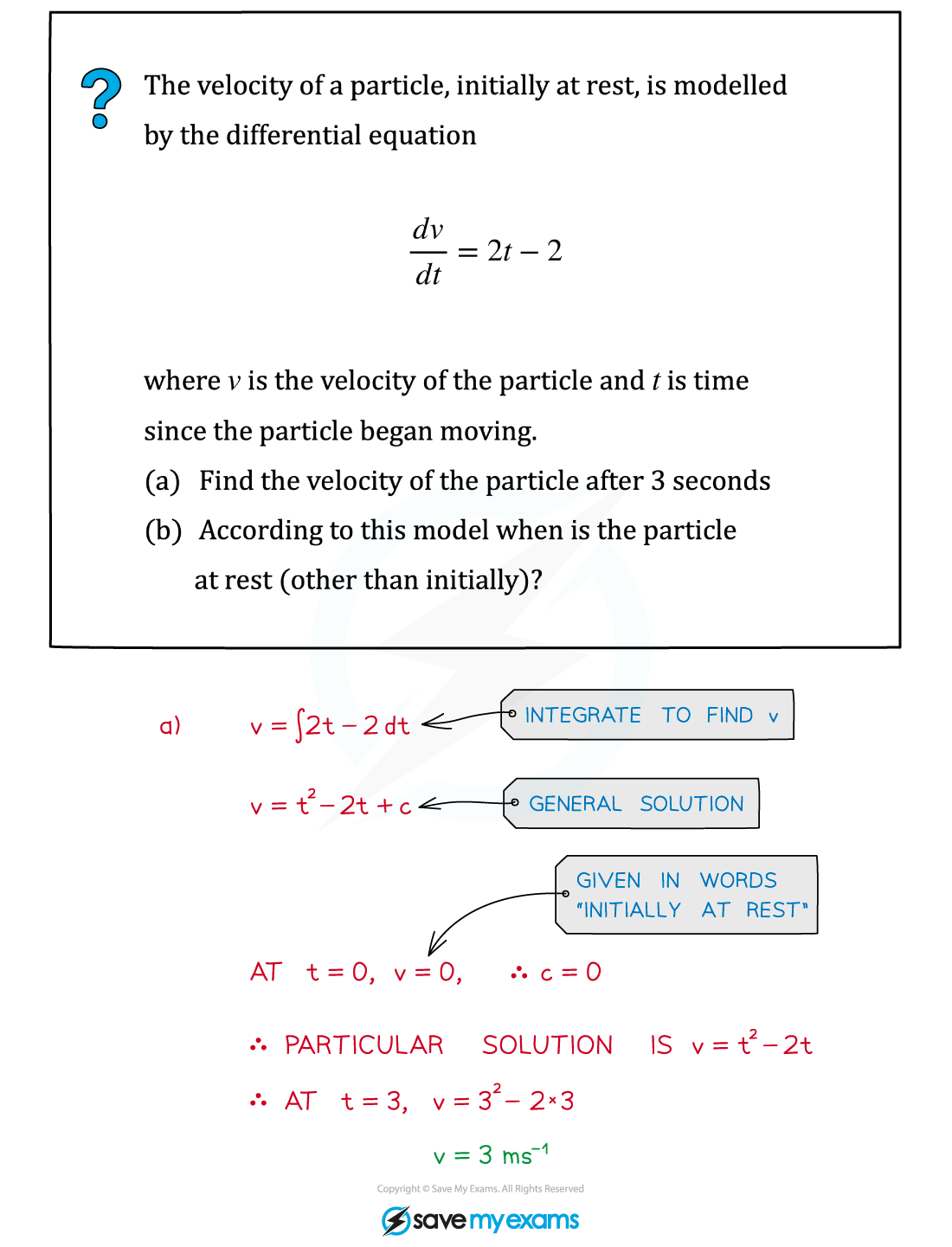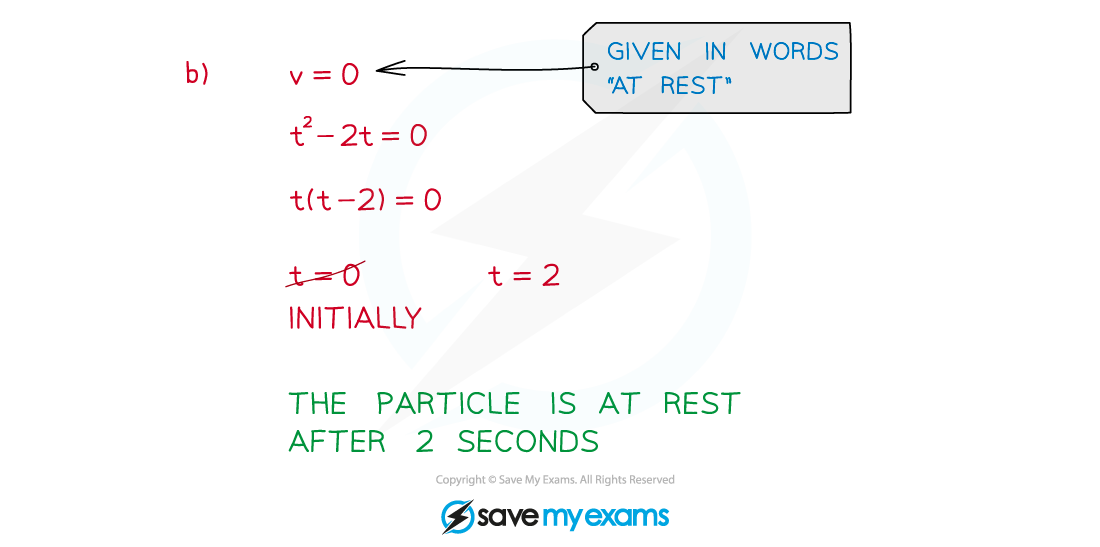# AQA A Level Maths: Pure复习笔记8.3.2 Particular Solutions

### Particular Solutions

#### What is a particular solution?

• Ensure you are familiar with General Solutions first
• With extra information, the constant of integration, c, can be found
• This means the particular solution (from the family of solutions) can be found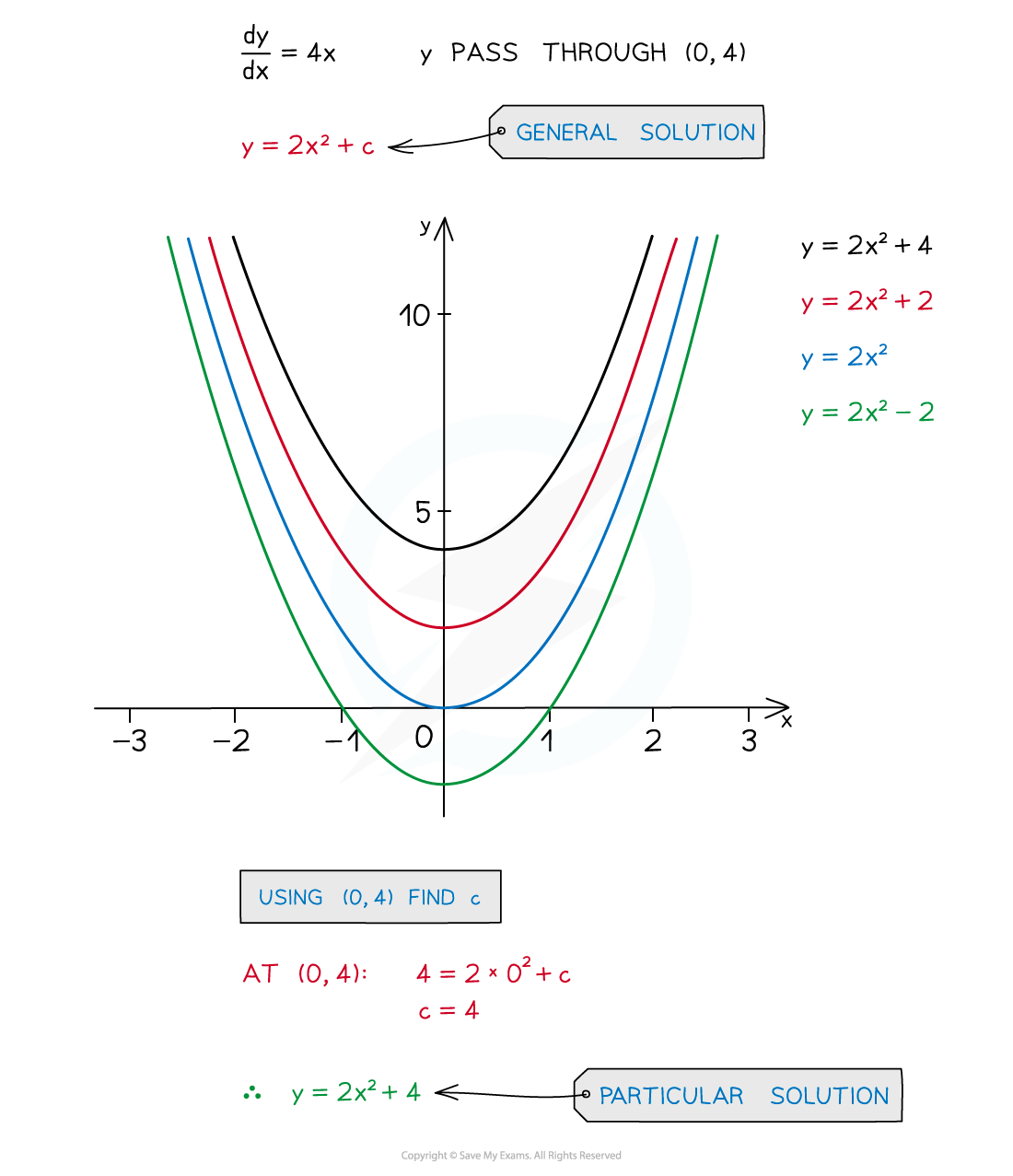#### What is a boundary condition/initial condition?

• A boundary condition is a piece of extra information that lets you find the particular solution
• For example knowing y = 4 when x = 0 in the preceding example
• In a model this could be a particle coming to rest after a certain time, ie v = 0 at time t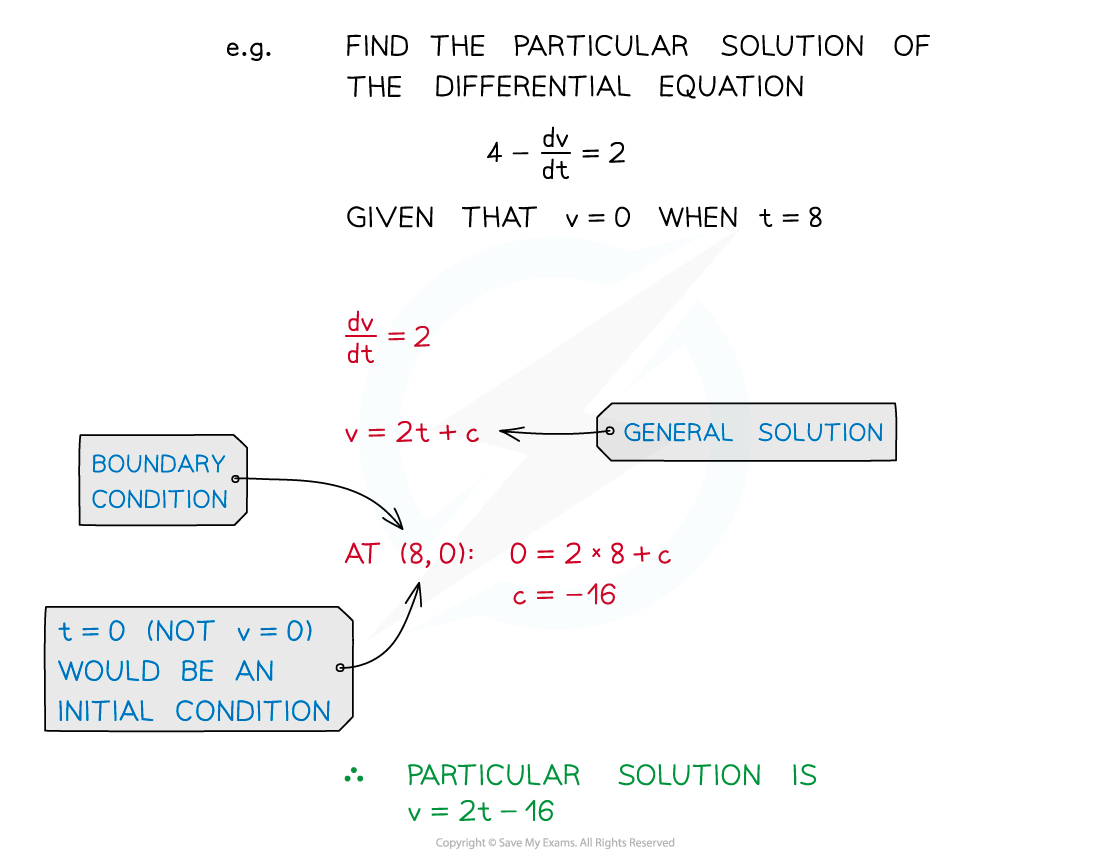• Differential equations are used in modelling, experiments and real-life situations
• A boundary condition is often called an initial condition when it gives the situation at the start of the model or experiment
• This is often linked to time, so t = 0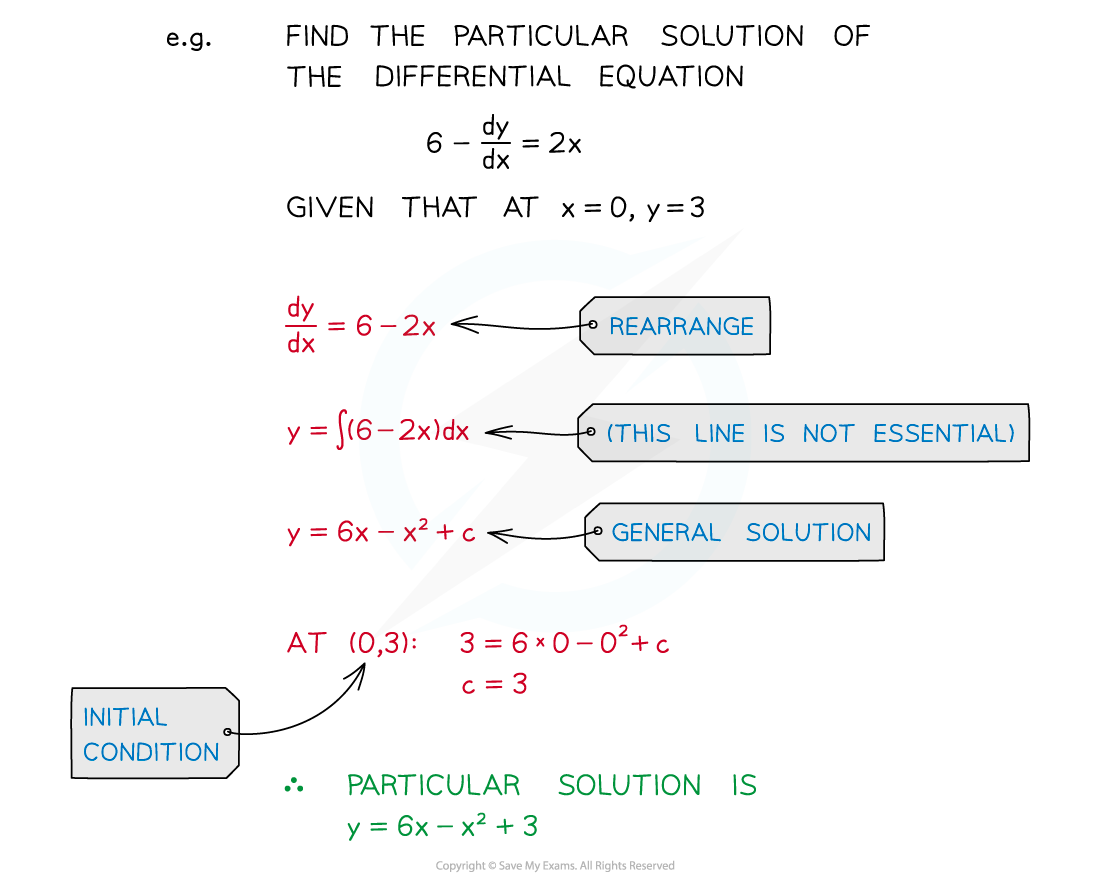• It is possible to have two boundary conditions
• eg a particle initially at rest has velocity, v = 0 and acceleration, a = 0 at time, t = 0
• for a second order differential equation you need two boundary conditions to find the particular solution

#### Worked Example